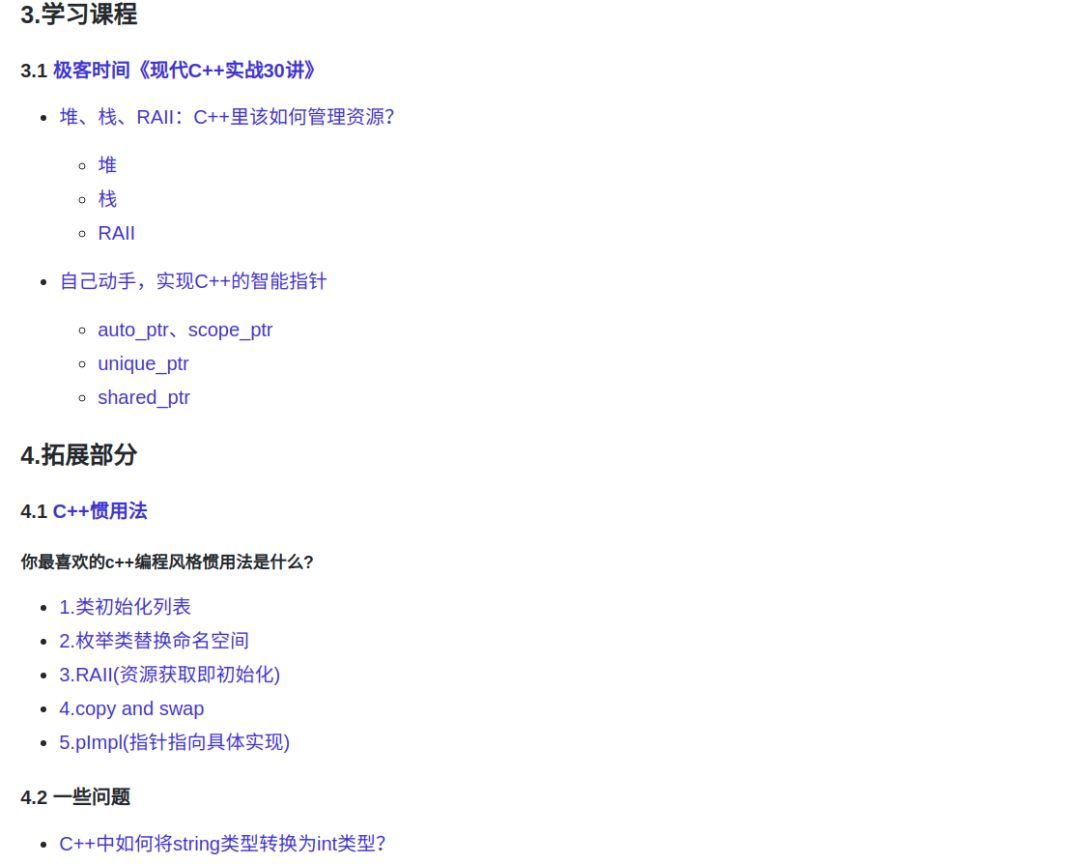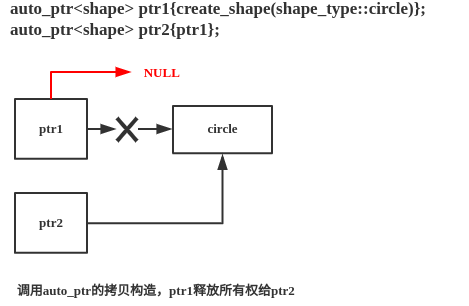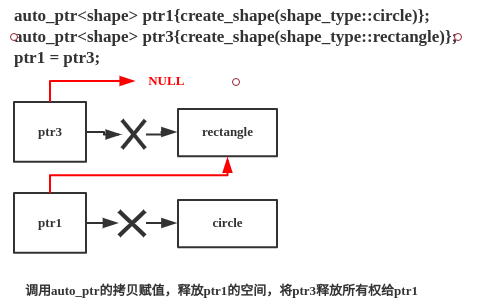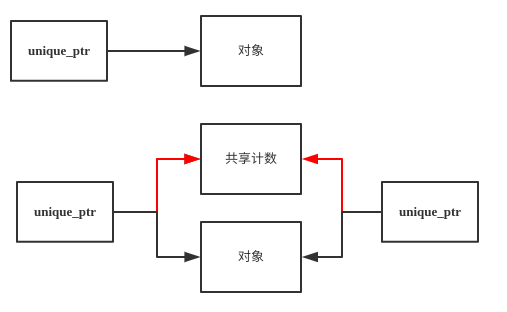[導讀]你最喜歡的C++編程風格慣用法是什麼？

## 0.回顧//github.com/Light-City/CPlusPlusThings

``class shape_wrapper {public:    explicit shape_wrapper( shape* ptr = nullptr) : ptr_(ptr) {}    ~shape_wrapper()    {        delete ptr_;    }    shape* get() const { return ptr_; }private:    shape* ptr_;};``

• 這個類只適用於 shape 類
• 該類對象的行為不夠像指針
• 拷貝該類對象會引發程序行為

## 1.手寫auto_ptr與scope_ptr

``template <typename  T>class smater_ptr {public:    explicit smater_ptr( T* ptr = nullptr) : ptr_(ptr) {}    ~smater_ptr()    {        delete ptr_;    }    T* get() const { return ptr_; }private:    T* ptr_;};``

``template <typename  T>class smater_ptr {public:   	...    T& operator*() const { return *ptr_; }    T* operator->() const { return ptr_; }    operator bool() const { return ptr_; }    ...private:    T* ptr_;};``

``smart_ptr ptr1{create_shape(shape_type::circle)};smart_ptr ptr2{ptr1};``

``template <typename T>class smart_ptr {    …    smart_ptr(const smart_ptr&)    = delete;    smart_ptr& operator=(const smart_ptr&)    = delete;    …};``

``template<typename T>class auto_ptr {public:    explicit auto_ptr( T *ptr = nullptr) noexcept : ptr_(ptr) {}    ~auto_ptr() noexcept {        delete ptr_;    }	// 返回值為T&，允許*ptr=10操作    T &operator*() const noexcept { return *ptr_; }    T *operator->() const noexcept { return ptr_; }    operator bool() const noexcept { return ptr_; }    T *get() const noexcept { return ptr_; }    // 拷貝構造,被複制放釋放原來指針的所有權,交給複製方    auto_ptr(auto_ptr &other) noexcept {        ptr_ = other.release();    }    // copy and swap    auto_ptr &operator=(auto_ptr &rhs) noexcept {// auto_ptr tmp(rhs.release());// tmp.swap(*this);        // s上述兩行等價於下面一行        auto_ptr(rhs.release()).swap(*this);        return *this;    }    // 原來的指針釋放所有權    T *release() noexcept {        T *ptr = ptr_;        ptr_ = nullptr;        return ptr;    }    void swap(auto_ptr &rhs) noexcept {        using std::swap;        swap(ptr_, rhs.ptr_);    // 轉移指針所有權    }private:    T *ptr_;};template<typename T>void swap(auto_ptr &lhs, auto_ptr &rhs) noexcept {    lhs.swap(rhs);}int main() {    auto_ptr ptr1{create_shape(shape_type::circle)};    auto_ptr ptr2{ptr1};    if (ptr1.get() == nullptr && ptr2.get())        cout << "拷貝構造：ptr1釋放了所有權,ptr2獲得了所有權" << endl;    ptr1 = ptr1;    auto_ptr ptr3{create_shape(shape_type::rectangle)};    ptr1 = ptr3;    if (ptr3.get() == nullptr && ptr1.get())        cout << "賦值操作：始終只有一個對象管理一個區塊!ptr3釋放了所有權,ptr1獲得了所有權" << endl;}``

``shapecircle拷貝構造：ptr1釋放了所有權,ptr2獲得了所有權shaperectangle賦值操作：始終只有一個對象管理一個區塊!ptr3釋放了所有權,ptr1獲得了所有權``

shape與circle實在create_shape時候輸出的，我們重點關注最後一句話，發現了一個很大的問題：它的行為會讓程序員非常容易犯錯。一不小心把它傳遞給另外一個 auto_ptr，你就不再擁有這個對象了。boost::scoped_ptr 屬於 boost 庫，定義在 namespace boost 中，包含頭文件`#include`可以使用。scoped_ptr 跟 auto_ptr 一樣，可以方便的管理單個堆內存對象，特別的是，scoped_ptr 獨享所有權，避免了auto_ptr惱人的幾個問題。

scoped_ptr是一種簡單粗暴的設計，它本質就是防拷貝，避免出現管理權的轉移。這是它的最大特點，所以他的拷貝構造函數和賦值運算符重載函數都只是聲明而不定義，而且為了防止有的人在類外定義，所以將函數聲明為private。但這也是它最大的問題所在，就是不能賦值拷貝，也就是説功能不全。但是這種設計比較高效、簡潔。沒有 release() 函數，不會導致先前的內存泄露問題。下面我也將模擬實現scoped_ptr的管理機制(實際上就是前面提到的禁止拷貝)：

``template<class T>class scoped_ptr // noncopyable{public:    explicit scoped_ptr(T *ptr = 0) noexcept : ptr_(ptr) {    }    ~scoped_ptr() noexcept {        delete ptr_;    }    void reset(T *p = 0) noexcept {        scoped_ptr(p).swap(*this);    }    T &operator*() const noexcept {        return *ptr_;    }    T *operator->() const noexcept {        return ptr_;    }    T *get() const noexcept {        return ptr_;    }    void swap(scoped_ptr &rhs) noexcept {        using std::swap;        swap(ptr_, rhs.ptr_);    }private:    T *ptr_;    scoped_ptr(scoped_ptr const &);    scoped_ptr &operator=(scoped_ptr const &);};template<typename T>void swap(scoped_ptr &lhs, scoped_ptr &rhs) noexcept {    lhs.swap(rhs);}``

scoped_ptr特點總結：

1）與auto_ptr類似，採用棧上的指針去管理堆上的內容，從而使得堆上的對象隨着棧上對象銷燬時自動刪除；

2）scoped_ptr有着更嚴格的使用限制——不能拷貝，這也意味着scoped_ptr不能轉換其所有權，所以它管理的對象不能作為函數的返回值，對象生命週期僅僅侷限於一定區間（該指針所在的{}區間，而std::auto_ptr可以）；

3）由於防拷貝的特性，使其管理的對象不能共享所有權，這與std::auto_ptr類似，這一特點使該指針簡單易用，但也造成了功能的薄弱。

## 2.手寫unique_ptr之子類向基類轉換

``template<typename T>class unique_ptr {public:    explicit unique_ptr( T *ptr = nullptr) noexcept : ptr_(ptr) {}    ~unique_ptr() noexcept {        delete ptr_;    }    T &operator*() const noexcept { return *ptr_; }    T *operator->() const noexcept { return ptr_; }    operator bool() const noexcept { return ptr_; }    T *get() const noexcept { return ptr_; }    unique_ptr(unique_ptr &&other) noexcept {        ptr_ = other.release();    }    // copy and swap 始終只有一個對象有管理這塊空間的權限    unique_ptr &operator=(unique_ptr rhs) noexcept {        rhs.swap(*this);        return *this;    }    // 原來的指針釋放所有權    T *release() noexcept {        T *ptr = ptr_;        ptr_ = nullptr;        return ptr;    }    void swap(unique_ptr &rhs) noexcept {        using std::swap;        swap(ptr_, rhs.ptr_);    // 轉移指針所有權    }private:    T *ptr_;};template<typename T>void swap(unique_ptr &lhs, unique_ptr &rhs) {    lhs.swap(rhs);}``

``int main() {    unique_ptr ptr1{create_shape(shape_type::circle)};// unique_ptr ptr2{ptr1}; // error    unique_ptr ptr2{std::move(ptr1)};    // ok    unique_ptr ptr3{create_shape(shape_type::rectangle)};// ptr1 = ptr3; // error    ptr3 = std::move(ptr1); // ok}``

（1）第一種：當我們只是在原先的移動構造上面添加`template `，此時情況是移動構造變為帶模板的移動構造，可以進行子類向基類轉換，但是與移動構造相關的，則調用的是默認移動構造，除非是子類向基類轉換，才調用帶模板的移動構造。

``template <typename U>unique_ptr(unique_ptr &&other) noexcept {    ptr_ = other.release();}``

• 默認構造函數，生成規則和C++98一樣，在用户沒有聲明自定義的構造函數的時候並且編譯期需要的時候生成。
• 析構函數，生成規則和C++98一樣，在C++11中有點不同的是，析構函數默認是noexcept。
• 拷貝構造函數，用户自定義了移動操作會導致不生成默認的拷貝構造函數，其它和C++98的行為一致。
• 拷貝賦值操作符，用户自定義了移動操作會導致不生成默認的拷貝賦值操作，其它和C++98的行為一致。
• 移動構造函數和移動賦值操作符，僅僅在沒有用户自定義的拷貝操作，移動操作和析構操作的時候才會生成。

``int main() {    unique_ptr ptr1{create_shape(shape_type::circle)};    unique_ptr ptr2{ptr1}; // 由於帶模板的移動構造函數引發編譯器會默認生成拷貝構造    if (ptr1.get() != nullptr)      // bitwise copy 此時ptr1不為NULL        ptr2.get()->print();    unique_ptr ptr2_2{std::move(ptr1)};    // 調用的是默認的移動構造,而不是帶模板的移動構造 bitwise move    if (ptr2_2.get() != nullptr && ptr1.get() != nullptr)   // ptr1 不為空        ptr2_2.get()->print();    unique_ptr ptr3{create_shape(shape_type::rectangle)};    ptr1 = ptr3;    // ok 根據形參先調用默認拷貝,再調用拷貝賦值    ptr3 = std::move(ptr1); // ok 根據形參先調用默認移動構造，而不是帶參數的移動構造,再調用移動賦值    unique_ptr ptr4(std::move(new circle));  // ok 調用帶模板的移動構造}``

（2）第二種：移動構造與帶模板的移動構造同時存在，可以完成子類向基類的轉換，此時是滿足上述生成規則，此時不會生成拷貝函數！

``int main() {    unique_ptr ptr1{create_shape(shape_type::circle)};// unique_ptr ptr2{ptr1}; // error    unique_ptr ptr2_2{std::move(ptr1)};    // ok    if (ptr2_2.get() != nullptr && ptr1.get() == nullptr)        ptr2_2.get()->print();    unique_ptr ptr3{create_shape(shape_type::rectangle)};// ptr1 = ptr3; // error    ptr3 = std::move(ptr1); // ok// unique_ptr cl{create_shape(shape_type::circle)}; // error 因為create_shape返回的是shape 不能基類轉子類    unique_ptr cl{new circle()};    unique_ptr ptr5(std::move(cl));  // ok unique轉unique}``

（1）我們需要了解子類向基類的隱式轉換，通過將移動構造函數變為帶模板的移動構造函數，要明白兩者共存情況與只有帶模板的移動或者其他構造函數對編譯器生成規則的影響！上述代碼，此時還不能完成基類向子類的轉換！例如:`unique_ptr``unique_ptr`

（2）auto_ptr與unique_tr都是獨佔所有權，每次只能被單個對象所擁有，unique_ptr與auto_ptr不同的是使用移動語義來顯示的編寫。auto_ptr是可以説你隨便賦值,但賦值完了之後原來的對象就不知不覺的報廢.搞得你莫名其妙。而unique_ptr就乾脆不讓你可以隨便去複製,賦值.如果實在想傳個值就哪裏,顯式的説明內存轉移std:move一下。然後這樣傳值完了之後,之前的對象也同樣報廢了.只不過整個move你讓明顯的知道這樣操作後會導致之前的unique_ptr對象失效。scope_ptr則是直接不允許拷貝。由於防拷貝的特性，使其管理的對象不能共享所有權

## 3.shared_ptr之引用計數

unique_ptr 算是一種較為安全的智能指針了。但是，一個對象只能被單個 unique_ptr所擁有，這顯然不能滿足所有使用場合的需求。一種常見的情況是，多個智能指針同時擁有一個對象；當它們全部都失效時，這個對象也同時會被刪除。這也就是 shared_ptr 了。``class shared_count {public:    shared_count() : count_(1) {    }    // 增加計數    void add_count() {        ++count_;    }    // 減少計數    long reduce_count() {        return --count_;    }    // 獲取當前計數    long get_count() const {        return count_;    }private:    long count_;};``

``template<typename T>class shared_ptr {public:    explicit shared_ptr( T *ptr = nullptr) noexcept : ptr_(ptr) {        if (ptr) {            shared_count_ = new shared_count();        }    }    ~shared_ptr() noexcept {        // 最後一個shared_ptr再去刪除對象與共享計數        // ptr_不為空且此時共享計數減為0的時候,再去刪除        if(ptr_&&!shared_count_->reduce_count()) {            delete ptr_;            delete shared_count_;        }    }	    void swap(shared_ptr &rhs) noexcept {        using std::swap;        swap(ptr_, rhs.ptr_);        swap(shared_count_,rhs.shared_count_);    }private:    T *ptr_;    shared_count *shared_count_;};template<typename T>void swap(shared_ptr &lhs, shared_ptr &rhs) noexcept {    lhs.swap(rhs);}``

``// copy and swap 始終只有一個對象有管理這塊空間的權限shared_ptr &operator=(shared_ptr rhs) noexcept {    rhs.swap(*this);    return *this;}``

``template<typename U>shared_ptr(const shared_ptr &other) noexcept {    ptr_ = other.ptr_;    if (ptr_) {        other.shared_count_->add_count();        shared_count_ = other.shared_count_;    }}template<typename U>shared_ptr(shared_ptr &&other) noexcept {    ptr_ = other.ptr_;    if (ptr_) {        shared_count_ = other.shared_count_;        other.shared_count_ = nullptr;    }}``

``‘circle* shared_ptr::ptr_’ is private``

``template<typename U>friend class shared_ptr;``

``long use_count() const noexcept {    if (ptr_) {        return shared_count_->get_count();    } else {        return 0;    }}``

``shared_ptr ptr1(new circle());cout << "use count of ptr1 is " << ptr1.use_count() << endl;shared_ptr ptr2, ptr3;cout << "use count of ptr2 was " << ptr2.use_count() << endl;ptr2 = ptr1;        // shared_ptr隱式轉換shared_ptr 調用帶模板的拷貝構造// cout<<"======="<// ptr3 = ptr2; // 調用的是編譯器生成的默認拷貝構造 所以引用計數不會增加 ptr3=ptr2// cout<<"======="<ptr3 = ptr1;cout << "此時3個shared_ptr指向同一個資源" << endl;cout << "use count of ptr1 is now " << ptr1.use_count() << endl;cout << "use count of ptr2 is now " << ptr2.use_count() << endl;cout << "use count of ptr3 is now " << ptr3.use_count() << endl;if (ptr1)    cout << "ptr1 is not empty" << endl;// 會先調用賦值函數,由編譯器決定調用的是拷貝構造還是移動構造,造出一個新的臨時對象出來,臨時對象會在跳出作用域後被析構掉。// 在析構函數中,會先判斷該臨時對象的是否指向資源,如果沒有,析構結束。否則,對引用計數減1,判斷引用計數是否為0,如果為0,刪除共享引用計數指針,否則不操作。cout << "此時2個shared_ptr指向同一個資源" << endl;ptr2 = std::move(ptr1);if (!ptr1 && ptr2) {      // 調用的是bool重載操作符    cout << "ptr1 move to ptr2" << endl;    cout << "use count of ptr1 is now " << ptr1.use_count() << endl;    cout << "use count of ptr2 is now " << ptr2.use_count() << endl;    cout << "use count of ptr3 is now " << ptr3.use_count() << endl;}``

``shapecircleuse count of ptr1 is 1use count of ptr2 was 0此時3個shared_ptr指向同一個資源use count of ptr1 is now 3use count of ptr2 is now 3use count of ptr3 is now 3ptr1 is not empty此時2個shared_ptr指向同一個資源ptr1 move to ptr2use count of ptr1 is now 0use count of ptr2 is now 2use count of ptr3 is now 2~circle~shape``

• 上述代碼沒有考慮線程安全性，這裏只是簡化版

• =賦值重載函數不加`&`，編譯器決定調用拷貝構造還是移動構造，來造出一個臨時對象出來。

• 根據前面提到的，當類中特殊函數變為帶模板的函數，編譯器仍然會生成默認拷貝構造與默認移動構造。

``shared_ptr ptr2, ptr3;ptr3 = ptr2;        // 調用的是編譯器生成的默認拷貝構造 所以引用計數不會增加``

## 4.指針類型轉換

• dynamic_cast
• static_cast
• const_cast
• reinterpret_cast

### 4.1 dynamic_cast

``// 實現強制類型轉換需要的構造函數template<typename U>shared_ptr(const shared_ptr &other, T *ptr) noexcept {    ptr_ = ptr;    if (ptr_) {        other.shared_count_->add_count();        shared_count_ = other.shared_count_;    }}``

``template<typename T, typename U>shared_ptr dynamic_pointer_cast(const shared_ptr &other) noexcept {    T *ptr = dynamic_cast(other.get());    return shared_ptr(other, ptr);}``

``// shape* -> circle* 使用dynamic_cast轉換後,指針為空.此時資源還是被dptr2擁有,dptr1為0shared_ptr dptr2(new shape);shared_ptr dptr1 = dynamic_pointer_cast(dptr2);      // 基類轉子類cout << "use count of dptr1 is now " << dptr1.use_count() << endl;    // 0cout << "use count of dptr2 is now " << dptr2.use_count() << endl;    // 1// circle* -> circle* 使用dynamic_cast轉換後,指針不為空,此時資源被兩者共同使用,引用計數為2shared_ptr dptr3(new circle);// shared_ptr dptr3(new circle); // 上面或者當前行,後面輸出一樣！shared_ptr dptr1_1 = dynamic_pointer_cast(dptr3);      // 基類轉子類cout << "use count of dptr1_1 is now " << dptr1_1.use_count() << endl;    // 2cout << "use count of dptr3 is now " << dptr3.use_count() << endl;    // 2// circle* -> circle* 使用dynamic_cast轉換後,指針不為空,此時資源被兩者共同使用,引用計數為2shared_ptr dptr3_1(new circle);shared_ptr dptr2_1 = dynamic_pointer_cast(dptr3_1);      // 子類轉基類 上行轉換,安全！cout << "use count of dptr2_1 is now " << dptr2_1.use_count() << endl;    // 2cout << "use count of dptr3_1 is now " << dptr3_1.use_count() << endl;    // 2``

dynamic_cast主要用於類層次間的上行轉換和下行轉換，還可以用於類之間的交叉轉換。在類層次間進行上行轉換時，dynamic_cast和static_cast的效果是一樣的；在進行下行轉換時，dynamic_cast具有類型檢查的功能，比static_cast更安全。在多態類型之間的轉換主要使用dynamic_cast，因為類型提供了運行時信息。

（1）下行轉換，基類轉換為子類，例如：智能指針轉換類似於`shape*` 轉換為`circle*` 使用dynamic_cast轉換後,指針為空.此時資源還是被dptr2擁有,dptr1為0。比static_cast安全。

（2）平行轉換，指向一致的相互轉換，例如：智能指針轉換類似於`circle*`轉換為`circle*`。此時引用計數為兩者共享。

（3）上行轉換，子類轉基類，例如：智能指針轉換類似於`circle*`轉換為`shape*`，此時引用技術為兩者共享。等價於static_cast。

### 4.2 static_cast

``template<typename T, typename U>shared_ptr static_pointer_cast(const shared_ptr &other) noexcept {    T *ptr = static_cast(other.get());    return shared_ptr(other, ptr);}``

``// shape* -> circle* 使用static_cast轉換後,指針為空  與dynamic_cast相比,不安全shared_ptr sptr2(new shape);shared_ptr sptr1 = static_pointer_cast(sptr2);      // 基類轉子類cout << "use count of sptr1 is now " << dptr1.use_count() << endl;    // 0cout << "use count of sptr2 is now " << dptr2.use_count() << endl;    // 1// circle* -> circle* 使用dynamic_cast轉換後,指針不為空,此時資源被兩者共同使用,引用計數為2shared_ptr sptr3(new circle);//    shared_ptr sptr3(new circle);     // 上面或者當前行,後面輸出一樣！shared_ptr sptr1_1 = static_pointer_cast(sptr3);      // 基類轉子類cout << "use count of sptr1_1 is now " << sptr1_1.use_count() << endl;    // 2cout << "use count of sptr3 is now " << sptr3.use_count() << endl;    // 2// circle* -> circle* 使用static_cast轉換後,指針不為空,此時資源被兩者共同使用,引用計數為2 等價於dynamic_castshared_ptr sptr3_1(new circle);shared_ptr sptr2_1 = static_pointer_cast(sptr3_1);      // 子類轉基類 上行轉換,安全！cout << "use count of sptr2_1 is now " << sptr2_1.use_count() << endl;    // 2cout << "use count of sptr3_1 is now " << sptr3_1.use_count() << endl;    // 2``

### 4.3 const_cast

``template<typename T, typename U>shared_ptr const_pointer_cast(        const shared_ptr &other) noexcept {    T *ptr = const_cast(other.get());    return shared_ptr(other, ptr);}``

``shared_ptr s = const_pointer_cast(shared_ptr<const circle>(new circle));``

### 4.4 reinterpret_cast

``template<typename T, typename U>shared_ptr reinterpret_pointer_cast(        const shared_ptr &other) noexcept {    T *ptr = reinterpret_cast(other.get());    return shared_ptr(other, ptr);}``

``int a = reinterpret_pointer_cast<int>(s);``••••## [嵌入式案例Show]STM32 時鐘分析

01 前言 在嵌入式系統中時鐘是其脈搏，處理器內核在時鐘驅動下完成指令執行，狀態變換等動作。外設部件在時鐘的驅動下完成各種工作，比如串口數據的發送、A/D轉換、定時器計數等等。...

## [嵌入式案例Show]常用的排序算法（C語言）

01 前言 排序是數據處理中經常運用的一種重要運算，排序的功能是將一個數據元素（記錄）的任意序列，重新排列成一個按照一個規則有序的序列。常用的排序算法我們要熟練掌握。 02...

## [嵌入式案例Show]嵌入式軟件中的延時函數

1、前言 延時函數是嵌入式軟件開發中必不可少的功能函數，在每個工程裏都能找到它的蹤影。雖然看起來不起眼，但在有些時序控制的場合，使用了一點點delay，往往能解決大問...